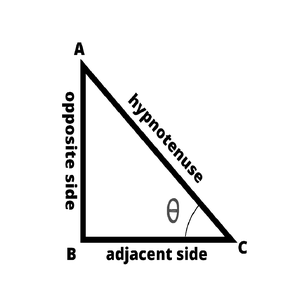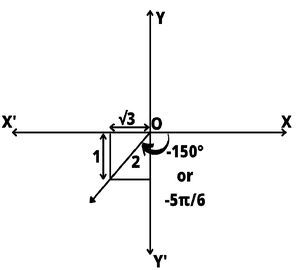Open in App
Not now

# How to find the value of tan(-150)°?

• Difficulty Level : Medium
• Last Updated : 15 Feb, 2022

Trigonometry is the branch of mathematics that deals with the ratios and the relations among the sides and angles in a triangle. Using trigonometry we can calculate various measurements connected to a triangle. Some standard ratios are defined for the ease of calculation of some common problems related to the length and angles of the sides of a right-angled triangle.

### Trigonometric Ratios

A trigonometric ratio is the proportion of sides with either of the acute angles in the right-angled triangle. We can define a simple trigonometric ratio in terms of sides of a right-angled triangle i.e. the hypotenuse, base side, and the perpendicular side. We have three simple trigonometric ratios wiz. sine, cosine, and tangent.Sine is the function that takes in the parameter an angle θ, which is either of the acute-angles in the right-angled triangles and is defined as the ratio of the length of the opposite side to the hypotenuse of the right-angled triangle. In technical terms, it can be written as,

sin(θ) = opposite side/hypotenuse

Cosine is the function that takes in the parameter an angle θ, which is either of the acute-angles in the right-angled triangles and is defined as the ratio of the length of the adjacent side to the hypotenuse of the right-angled triangle. In technical terms, it can be written as,

Tangent is the function that takes in the parameter an angle θ, which is either of the acute-angles in the right-angled triangles and is defined as the ratio of the length of the opposite side to the adjacent side of the right-angled triangle. In technical terms, it can be written as,

tan(θ) = opposite side / adjacent side

These trigonometric  ratios are related to one another using some trigonometric identities and formulas,

tan(θ) = sin(θ) / cos(θ)

sin2(θ) + cos2(θ) = 1

Each of the trigonometric ratios has other three derived trigonometric ratios which are deduced by taking the inverse of the respective ratios. The Other three Trigonometric ratios are Cosecant, Secant, and Cotangent used mathematically as cosec, sec, and cot. These are related to the Primary trigonometric ratios as follows,

cosec(θ) = 1 / sin(θ)

sec(θ) = 1 / cos(θ)

cot(θ) = 1 / tan(θ) = cos(θ) / sin(θ)

Below are some of the identities related to the standard trigonometric ratios and the derived trigonometric ratios,

tan2(θ) + 1 = sec2(θ)

cot2(θ) + 1 = cosec2(θ)

Trigonometric Table

The below is the table for some common angles and the basic trigonometric ratios.

There are also some other trigonometric ratios to apply beyond the right-angled triangles:

sin(-θ) = – sin(θ)

cos(-θ) = cos(θ)

tan(-θ) = – tan(θ)

Special trigonometric formula for tangent function,

tan (A + B) = (tan(A) + tan(B))/(1 – (tan(A).tan(B)))

tan (A – B) = (tan(A) – tan(B))/(1 + (tan(A).tan(B)))

### What is the value of tan(-150)°?

A couple of methods can be used to calculate the value of tan(-150) by using various trigonometric identities and formulas.

Method 1

Make use of various trigonometric identities and rules to calculate the value of tan(-150)°. Here use of the following identities and formulas,

tan(θ) = sin(θ)/cos(θ)

tan(-θ) = -tan(θ)

tan(180 + θ) = tan(θ), or

tan(180 – θ) = -tan(θ)

Solution:

tan(-150)

tan(-θ) = -tan(θ)

here, θ = 150

tan(-150) = – tan(150)

Now, by using the Trigonometrical ratios of (n180 – θ),

tan( -150 ) = – tan(180 – 30)

tan(180 – θ) = -tan(θ)

tan(-150) = -(- tan (30))

= tan(30)

= 1/√3

Therefore tan(-150)° = 1/√3

= 0.57735.

Method 2

Make use of the cartesian coordinate system to calculate the value of tan(-150). Here the use of the following identities and formulas are done,

tan(θ) = y/x (where x and y are the coordinates of the points on the unit circle)In a unit circle,

So, take the Y-coordinate as 1,

Using the Cartesian- Coordinate system,

Find the angle subtended by X-axis and the angle (-150)

We can calculate the angle = (180 -150) = 30

Thus the triangle formed is a 30-60-90 triangle,

Using the properties of the 30-60-90 triangle, we can find the rest of the sides,

The side adjacent to the angle is √3 times the opposite side.

And, the hypotenuse is 2 times the opposite side.

Thus, the opposite side = 1 unit,

And hypotenuse becomes = 2 units.

Thus, by definition,

tan(θ) = opposite side / adjacent side

So, tan(-150) = 1/√3

Method 3

We can convert the tangent function to sine and cosine functions for simplifying the calculation of tan(-150). Here we make use of the following identities and formulas,

tan(θ) = sin(θ)/cos(θ)

sin(-θ) = -sin(θ)

cos(-θ) = cos(θ)

sin(90 + θ) = cos(θ)

cos(90 + θ) = -sin(θ)

Solution:

tan(θ) = sin(θ)/cos(θ)

Here, θ = -150

tan(-150) = sin(-150) / cos(-150)

sin(-θ) = -sin(θ) and cos(-θ) = cos(θ)

tan(-150) = -sin(150) / cos(150)

Convert 150 as 90 + 60,

tan(-150) = -sin(90+60) / (cos(90+60)

Since, sin(90 + θ) = cos(θ) and cos(90 + θ) = -sin(θ)

tan(-150) = -(cos(60)) / (-sin(60)

tan(-150) = cos(60) / sin(60)

= (1/2) / (√3/2)       Since cos(60) = 1/2 and sin(60) = √3/2

= 1/√3

Therefore,

tan(-150) = 1/√3

Method 4

Use some of the tangent functions for the calculation of tan(-150). Here we make use of the following identities and formulas,

tan(-θ) = -tan(θ)

tan(A-B) = (tan(A) – tan(B)) / (1 + (tan(A).tan(B)))

So, by using the above identities and relations we can deduce the value of tan(-150)

tan(-150)

By using tan(-θ) = -tan(θ),

tan(-150) = -tan(150)

Now,

tan(-150) = -tan( 180 – 30)

By using, tan(A-B) = (tan(A) – tan(B)) / (1 + (tan(A).tan(B)))

Where, A = 180 and B=30,

tan(-150) = – [tan(180) -tan(30)] / [1 + (tan(180).tan(30))]

= – [0 – 1/√3] / [1 + (0.1/√3)]

= – [-1/√3] / [1 + 0]

= 1/√3

Therefore,

tan(-150) = 1/√3

Thus, from the following methods, we were able to find the value of tan(-150) or tan(-5pi/6) as 1/√3 which is approximately 0.57735.

### Similar Problems

Question 1: If sin(A) = 4/5 what is tan(A)?

Solution:

sin(A) = 4/5

sin(θ) = opposite side / hypotenuse

So, here,

Opposite side = 4 units and hypotenuse = 5 units

By Pythagoras Theorem, we can calculate Adjacent sides’ length.

(Hypotenuse side)2 = (opposite side)2 + (adjacent side)2

Therefore,

(Adjacent side)2  = (hypotenuse)2 – (opposite side)2

(Adjacent side)2  = (5)2 – (4)2

= 25 – 16 = 9

tan(θ) = opposite side / adjacent side

tan(A) = 4/3

Question 2: Find the value of sin(120).

Solution:

sin(120) = sin(90 + 30)

Therefore, by applying the relation,

sin(90 + θ) = cos(θ),

sin(120) = sin(90 + 30)

= cos(30)

= √3/2

Question 3: Find the value of tan(120)

Solution:

tan(120) = tan(180 – 60)

Therefore, by applying the relation,

tan(180 – θ) = -tan(θ),

tan(120) = tan(180 – 60)

= -tan(60)

= -√3      Since tan(60) = √3

Therefore,

tan(120) = √3

My Personal Notes arrow_drop_up
Related Articles